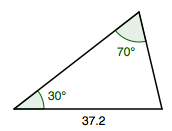### Home > PC3 > Chapter 12 > Lesson 12.1.4 > Problem12-71

12-71.

One angle of a triangle measures $70º$ and the opposite side has a length of $37.2$ feet. Another angle of the triangle measures $30º$. Solve the triangle and calculate its area.

The sum of the angles in a triangle is $180º$.

Use the Law of Sines to calculate the remaining side lengths.

$\frac{\text{sin}(70º)}{37.2}=\frac{\text{sin}(30º)}{x}$